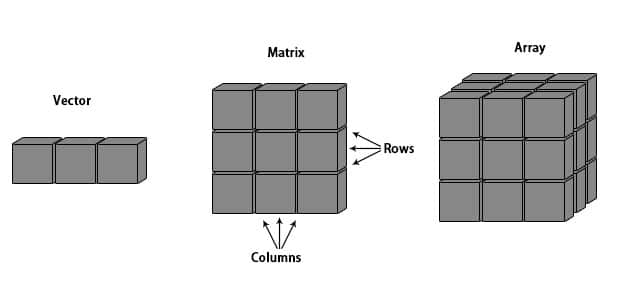# blog posts# Arrays are data objects in the R programming language that can store data in more than two dimensions. For example – if we create an array of dimensions (2,3,4);

This array can then create 4 rectangular matrices, each with 2 rows and 3 columns. Arrays can only store data types.

An array is created using the Array () function. This array adopts vectors as input and to create arrays; Uses values ​​that are dim parameters .

## Example

The following example creates an array of 3 matrices, each with 3 rows and 3 columns.

# Create two vectors of different lengths.

vector1 <- c (5,9,3)

vector2 <- c (10,11,12,13,14,15)

# Take these vectors as input to the array.

result <- array (c (vector1, vector2), dim = c (3,3,2))

print (result)

When we run the above code; The following results are generated:

,, ۱

[, 1] [, 2] [, 3]

[1,] 5 10 13

[2,] 9 11 14

[3,] 3 12 15

,, ۲

[, 1] [, 2] [, 3]

[1,] 5 10 13

[2,] 9 11 14

[3,] 3 12 15

## Names of rows and columns

We can arrange rows, columns and matrices within an array; Assign the desired name using the dimnames parameter .

# Create two vectors of different lengths.

vector1 <- c (5,9,3)

vector2 <- c (10,11,12,13,14,15)

column.names <- c (“COL1 ″,” COL2 ″, ”COL3”)

row.names <- c (“ROW1 ″,” ROW2 ″, ”ROW3”)

matrix.names <- c (“Matrix1 ″,” Matrix2 ”)

# Take these vectors as input to the array.

result <- array (c (vector1, vector2), dim = c (3,3,2), dimnames = list (row.names, column.names,

matrix.names))

print (result)

When we run the above code; The following results are generated:

,, Matrix1

COL1 COL2 COL3

ROW1 5 10 13

ROW2 9 11 14

ROW3 3 12 15

,, Matrix2

COL1 COL2 COL3

ROW1 5 10 13

ROW2 9 11 14

ROW3 3 12 15

## Access array members

# Create two vectors of different lengths.

vector1 <- c (5,9,3)

vector2 <- c (10,11,12,13,14,15)

column.names <- c (“COL1 ″,” COL2 ″, ”COL3”)

row.names <- c (“ROW1 ″,” ROW2 ″, ”ROW3”)

matrix.names <- c (“Matrix1 ″,” Matrix2 ”)

# Take these vectors as input to the array.

result <- array (c (vector1, vector2), dim = c (3,3,2), dimnames = list (row.names,

column.names, matrix.names))

# Print the third row of the second matrix of the array.

print (result [3,, 2])

# Print the element in the 1st row and 3rd column of the 1st matrix.

print (result [1,3,1])

# Print the 2nd Matrix.

print (result [,, 2])

When we run the above code; The following result is obtained:

COL1 COL2 COL3

۳ ۱۲ ۱۵

 13

COL1 COL2 COL3

ROW1 5 10 13

ROW2 9 11 14

ROW3 3 12 15

## Employ array members

As arrays are made of matrices with different dimensions; Operations on elements are performed by accessing matrix members.

# Create two vectors of different lengths.

vector1 <- c (5,9,3)

vector2 <- c (10,11,12,13,14,15)

# Take these vectors as input to the array.

array1 <- array (c (vector1, vector2), dim = c (3,3,2))

# Create two vectors of different lengths.

vector3 <- c (9,1,0)

vector4 <- c (6,0,11,3,14,1,2,6,9)

array2 <- array (c (vector1, vector2), dim = c (3,3,2))

# create matrices from these arrays.

matrix1 <- array1 [,, 2]

matrix2 <- array2 [,, 2]

result <- matrix1 + matrix2

print (result)

When we run the above code; We get the following result:

[, 1] [, 2] [, 3]

[1,] 10 20 26

[2,] 18 22 28

[3,] 6 24 30

## Computations on array members

Using the apply () function,  we can perform calculations on members of an array.

Syntax

apply (x, margin, fun)

The parameters that we write in the above code are as follows:

• x is an array.
• margin is the name of the data set used.
• fun is a function that must be applied to members of an array.

Example

In the following coding, we use the apply () function to calculate the sum of the elements in the rows of all the matrices in an array.

# Create two vectors of different lengths.

vector1 <- c (5,9,3)

vector2 <- c (10,11,12,13,14,15)

# Take these vectors as input to the array.

new.array <- array (c (vector1, vector2), dim = c (3,3,2))

print (new.array)

# Use apply to calculate the sum of the rows across all the matrices.

result <- apply (new.array, c (1), sum)

print (result)

When we run the above code; We achieve the following results:

,, ۱

[, 1] [, 2] [, 3]

[1,] 5 10 13

[2,] 9 11 14

[3,] 3 12 15

,, ۲

[, 1] [, 2] [, 3]

[1,] 5 10 13

[2,] 9 11 14

[3,] 3 12 15

 56 68 60